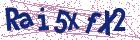# A robust uniformly convergent scheme for two parameters singularly perturbed parabolic problems with time delay

Document Type : Research Article

Author

Department of Mathematics, Wollega University, Nekemte, Ethiopia.

Abstract

A singularly perturbed time delay parabolic problem with two small pa-rameters is considered. The paper develops a finite difference scheme that is exponentially fitted on a uniform mesh in the spatial direction and uses the implicit-Euler method to discretize the time derivative in the temporal direction in order to obtain a better numerical approximation to the solu-tions of this class of problems. We establish the parameter-uniform error estimate and discuss the stability of the suggested approach. In order to demonstrate the improvement in terms of accuracy, numerical results are also shown to validate the theoretical conclusions and are contrasted with the current hybrid scheme.

Keywords

Main Subjects

#### References

 Asl, F.M., and Ulsoy, A.G. Analysis of a system of linear delay differ-ential equations, J. Dyn. Sys., Meas., Control, 125 (2) (2003), 215–223.
 Clavero, C., Jorge, J. and Lisbona, F. A uniformly convergent scheme on a nonuniform mesh for convection-diffusion parabolic problems. J. Comput. Appl. Math. 154(2) (2003), 415–429.
 Das, A. and Natesan, S. Uniformly convergent hybrid numerical scheme for singularly perturbed delay parabolic convection-diffusion problems on Shishkin mesh, Appl. Math. Comput. 271 (2015), 168–186.
 Epstein, I.R. Delay effects and differential delay equations in chemical kinetics, Int. Rev. Phys. Chem. 11 (1) (1992), 135–160.
 Govindarao, L., Sahu, S.R. and Mohapatra, J. Uniformly convergent numerical method for singularly perturbed time delay parabolic problem with two small parameters, Iran. J. Sci. Technol. Trans. A Sci. 43(5) (2019), 2373–2383.
 Gowrisankar, S. and Natesan, S. ε- uniformly convergent numerical scheme for singularly perturbed delay parabolic partial differential equa-tions, Int. J. Comput. Math. 94 ( 2017), 902–921.
 Kumar, D. A parameter-uniform scheme for the parabolic singularly perturbed problem with a delay in time, Numer. Methods Partial Differ. Equ. 37 (1) (2021), 626–642.
 Kumar, S. and Kumar, M. A robust numerical method for a two-parameter singularly perturbed time delay parabolic problem, Comput. Appl. Math. 39(3) (2020), 1–25.
 Ladyzhenskaia, O.A., Solonnikov, V.A. and Ural’tseva, N.N. Linear and quasilinear equations of parabolic type.(Russian) Translated from the Russian by S. Smith Translations of Mathematical Monographs, Vol. 23 American Mathematical Society, Providence, R.I. 1968.
 McCartin, B.J. Discretization of the semiconductor device equa-tions,”New problems and new solutions for device and process mod-eling”, Boole, (1985), 72–82.
 Miller, J., O’Riordan, E., Shishkin, G. and Shishkina, L. Fitted mesh methods for problems with parabolic boundary layers, Math. Proc. R. Ir. Acad. 98A (1998), no. 2, 173–190.
 Negero, N.T. A uniformly convergent numerical scheme for two param-eters singularly perturbed parabolic convection-diffusion problems with a large temporal lag, Results Appl. Math. 16 (2022), Paper No. 100338, 15pp.
 Negero, N.T. and Duressa, G.F. A method of line with improved accuracy for singularly perturbed parabolic convection-diffusion problems with large temporal lag, Results Appl. Math. 11 (2021), 100174, 13 pp.
 Negero, N.T. and Duressa, G.F. An efficient numerical approach for singularly perturbed parabolic convection-diffusion problems with large time-lag, J. Math. Model. 10(2) (2022), 173–110.
 Negero, N.T. and Duressa, G.F. Uniform convergent solution of singu-larly perturbed parabolic differential equations with general temporal-lag, Iran. J. Sci. Technol. Trans. A Sci. 46(2) (2022), 507–524.
 Negero, N.T. and Duressa, G.F. An exponentially fitted spline method for singularly perturbed parabolic convection-diffusion problems with large time delay, Tamkang J. Math. (2022).
 Negero, N.T. and Duressa, G.F. Parameter-uniform robust scheme for singularly perturbed parabolic convection-diffusion problems with large time-lag, Comput. Methods Differ. Equ. 10 (4) (2022), 954–968.
 O’Malley Jr, R.E. Introduction to singular perturbations, Applied Math-ematics and Mechanics, Vol. 14. Academic Press [Harcourt Brace Jo-vanovich, Publishers], New York-London, 1974.
 Roos, H.G. and Uzelac, Z. The SDFEM for a convection-diffusion prob-lem with two small parameters, Dedicated to John J. H. Miller on the oc-casion of his 65th birthday. Comput. Methods Appl. Math. 3(3) (2003),443–458.
 Tikhonov, A.N. and Samarskii, A.A. Equations of mathematical physics, Courier Corporation, 2013.
 Van Harten, A. and Schumacher, J. On a class of partial functional dif-ferential equations arising in feed-back control theory, Differential equa-tions and applications (Proc. Third Scheveningen Conf., Scheveningen, (1977), pp. 161–179, North-Holland Math. Stud., 31, North-Holland, Amsterdam-New York, 1978.
 Woldaregay, M. M. Aniley, W. T. and Duressa,G.F. Novel numerical scheme for singularly perturbed time delay convection-diffusion equation Adv. Math. Phys. (2021), Art. ID 6641236, 13 pp.
 Wu, J. Theory and applications of partial functional differential equa-tions, New York, Springer, 119, (2012).